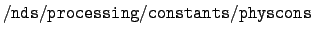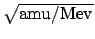Next: Code details for mcfgen Up: The format for mcf.bin' Previous: Target directory   Contents

## Overall directory

The overall directory starts with 23 entries giving basic information about the file. Then comes information about the possible secondary particles. The energy groups are given next, followed by the the target temperature and a list of physical constants. The overall directory ends with the identification and location of each target directory. The specific content of the overall directory is as follows.

0:
An mcf.bin' file always has a zero as the first entry. It is therefore convenient to start the count so that item number 1 is the first nonzero entry.

1:
The length of the overall directory.

2:
Identification of the incident particle. See Section 2.1.

3:
Atomic number and mass number for the incident particle, coded as 1000*Z + A.

4:
The atomic mass of the incident particle.

5:
The halflife of the incident particle. Stable particles are given a halflife of 1050.

6:
The number of targets in this file.

7:
The date of the library. Here, 21 November 1994 is coded as 941121.

8:
The number of energy group bounds.

9:
The location in this directory of the energy group bounds.

10:
The number of temperatures. This number must be 1, because Doppler broadening is not done in mcfgen.

11:
The location in this directory of the temperatures.

12:
The number of physical constants.

13:
The location in this directory of the physical constants.

14:
The maximum size of a block of target data.

15:
The interpolation type. This is 0 for linear-linear interpolation and 5 for log-log.

16:
The length of a structure for identifying and locating a target directory. This number is currently 5.

17:
The location in this directory of the first structure for identifying and locating a target directory.

18:
The number of additional directory items. In fact, this number is 22, and these items identify the possible secondary particles.

19:
The location of these additional directory items.

20:
The amount of space in this directory devoted to structures identifying and locating target directories. It is the product of items 6 and 16.

21:
A length once associated with target directories. Its value is 6, but it is not used.

22:
The library type. This is 0 for group-averaged data and 1 for grid-based data.

Next comes the 22 additional items referred to in items 18 and 19 above.

Number of possible secondary particles:
This number is now 7.

The particle identifiers:
The numbers 1-7 for neutron, proton, deuteron, triton. 3He, alpha, and photon.

Atomic masses:
The next seven numbers are the atomic masses of the above particles.

ZA numbers:
There follows the seven values of 1000*Z + A for the possible secondary particles.

Next comes the energy groups as mentioned in items 8 and 9 above. These are followed by the target temperature, and this location is given in item 11 in the overall directory. Then come the physical constants referred to in items 12 and 13. These constants are copied from the default bdfls' fileon the Nuclear Data Groups' network, and they are defined in that file. At the present time these constants are
1.
A conversion factor, erg/Mev.

2.

3.
Conversion factor, sec**2/sh**2.

4.
Conversion factor, (cm/sh)*, may be used to convert particle energy to velocity in Newtonian mechanics.

5.
Conversion factor, Mev/amu.

6.
Conversion factor, jerks/kton, where kton = 1012 cal.

7.
Conversion factor, Mev/jerk, where jerk = 1016 ergs or 109 joules.

8.
Speed of light in vacuum in units of cm/sh.

9.
Planck's constant in units of Mev sh.

10.
Boltzmann's constant in units of Mev/K.

11.
Rest mass of the electron in amu.

The overall directory ends with a collection of the following structures, one for each target.

Target ZA:
The atomic number and mass number of the target, coded as 1000*Z + A.

Target Z:
The atomic number of the target.

Atomic mass:
The atomic mass of the target.

Target directory location:
The location of the target directory in this file.

Target directory length:
The length of the target directory.Next: Code details for mcfgen Up: The format for mcf.bin' Previous: Target directory   Contents